﻿ py征途3之填坑（pagerank个人详解）

py征途3之填坑（pagerank个人详解）

2021-12-9 20:52:39

py征途这个坑也是挖了许久未填的大坑，其实一直以来都有学习，甚至还做了几个小项目，这里就小宣传一波：
github的项目说明及源码这次的pagerank就放到csdn吧。（主要是懒，不想再去写readme）

(不得不说，数学公示在markdown中是一生之敌，就用C代码的代码块勉强表示吧，需要的小伙伴可以下载并查看pdf哦。)

PageRank的算法研究

一，数学原理

（一）矩阵

𝑎11 𝑎12 ⋯ 𝑎1𝑛
𝑎21 𝑎22 ⋯ 𝑎2𝑛
⋮ ⋮ ⋮
𝑎𝑚1 𝑎𝑚2 ⋯ 𝑎𝑚𝑛

A=(
𝑎11 𝑎12 ⋯ 𝑎1𝑛
𝑎21 𝑎22 ⋯ 𝑎2𝑛
⋮ ⋮
𝑎𝑚1 𝑎𝑚2 ⋯ 𝑎𝑚𝑛
)

2 矩阵的计算

（1）加法

（𝑖） A +B = B +A；
（𝑖𝑖）（A +B）+ C = A +（B +C）

（2）数与矩阵相乘

λA==(
𝜆𝑎11 𝜆𝑎12 ⋯ 𝜆𝑎1𝑛
𝜆𝑎21 𝜆𝑎22 ⋯ 𝜆𝑎2𝑛
⋮ ⋮
𝜆𝑎𝑚1 𝜆𝑎𝑚2 ⋯ 𝜆𝑎𝑚𝑛
)

（𝑖）（λμ）A =λ（μA）
（𝑖𝑖）（λ+μ）A =λA +μA
（𝑖𝑖𝑖）λ（A +B）=λA +λB

（3）矩阵与矩阵的乘法

𝐶𝑖𝑗 = 𝑎𝑖1𝑏1𝑗 + 𝑎𝑖2𝑏2𝑗 ++ 𝑎𝑖𝑠𝑏𝑠𝑗 = ∑𝑎𝑖𝑘𝑏𝑘𝑗 (𝑖 = 1,2,, 𝑚;𝑗 = 1,2,, 𝑛)

（𝑖）（A B）C = A（B C）
（𝑖𝑖）λ（A B）=（λA）B = A（λB）（其中λ为数）
（𝑖𝑖𝑖） A（B + C）= A B +A C，（B + C）A = BA + CA

（4）矩阵的转置

（𝑖）(𝐴𝑇)𝑇 = A
（𝑖𝑖）(𝐴 + 𝐵)𝑇= 𝐴𝑇 +𝐵𝑇
（𝑖𝑖𝑖） (𝜆𝐴)𝑇= 𝜆𝐴𝑇
（𝑖𝑣）(𝐴 𝐵)𝑇 = 𝐴𝑇𝐵𝑇

（二）一阶马尔可夫链

P(𝑋𝑡|𝑋0,𝑋1,, 𝑋𝑡−1) = P(𝑋𝑡+1|𝑋𝑡)t = 0,1,2,

（三）马尔可夫模型

⋯ 𝑋𝑡−2,𝑋𝑡−1,𝑋𝑡,𝑋𝑡+1 ⋯，那么我们的在时刻 Xt+1 的状态的条件概率仅仅依赖于时刻𝑋𝑡 ，即：

P(𝑋𝑡+1|, 𝑋𝑡−2,𝑋𝑡−1,𝑋𝑡) = P(𝑋𝑡+1|𝑋𝑡)

二、PageRank 定义

（一） 基本思想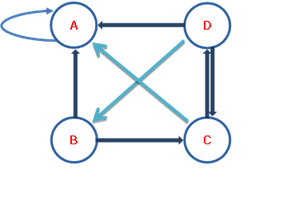PageRank 值依赖于网络的拓扑结构，一旦网络的拓扑(连接关系)确定，
PageRank 值就确定。

（二）有向图和随机游走模型

1 有向图

（strongly connected graph） 。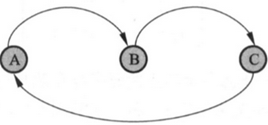2 随机游走模型

𝑀 = [𝑚𝑖𝑗]𝑚×𝑛

𝑚𝑖𝑗 ≥ 0∑𝑚𝑖𝑗𝑛𝑖=1

（3）随机游走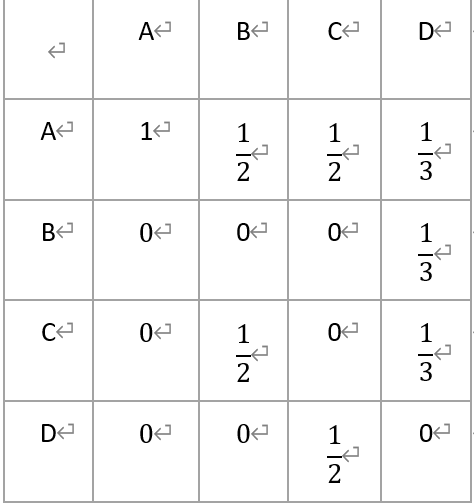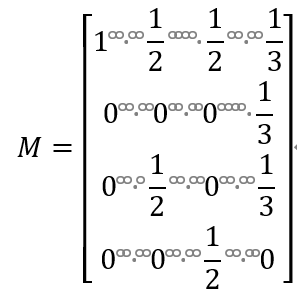𝑅𝑡+1 = 𝑀𝑅𝑡

𝑅0, 𝑀𝑅0, 𝑀2𝑅0, ⋯ , 𝑀𝑡𝑅0则极限lim𝑡→∞𝑀𝑡𝑅0 = 𝑅存在，极限向量 R 表示马尔可夫链的平稳分布，满足MR=R。

（三）PageRank 的基本定义

2 具体定义

PageRank 值。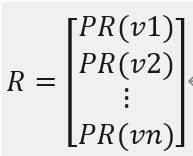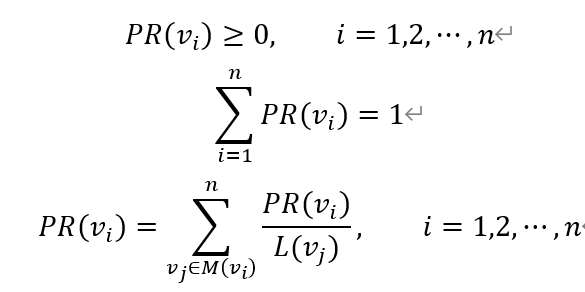M(vi)表示指向结点𝑣𝑖的结点集合。
L(𝑣𝑗)表示结点𝑣𝑗连出的有向边的个数。
PageRank 的基本定义是理想化的，在这中情况下，PageRank 存在，而且可以通过不断迭代求得 PageRank 值。

三、案例分析

（二）状态转移矩阵和初始分布向量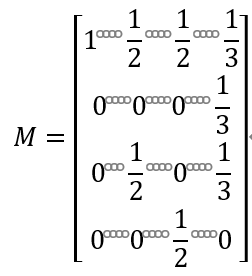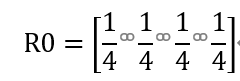（三）进行迭代运算

1 单步矩阵相乘

import numpy as np
a=np.array([[1,1/2,1/2,1/3],[0,0,0,1/3],[0,1/2,0,1/3],[0,0,1/2,0]])
b=np.array([1/4,1/4,1/4,1/4])
print(np.dot(a,b))

2 设计迭代相乘

import numpy as np
a=np.array([[1,1/2,1/2,1/3],[0,0,0,1/3],[0,1/2,0,1/3],[0,0,1/2,0]])
b=np.array([1/4,1/4,1/4,1/4])
while 1:
PR=np.dot(a,b)
print(PR)
b=PR

R0 = [1 0 0 0]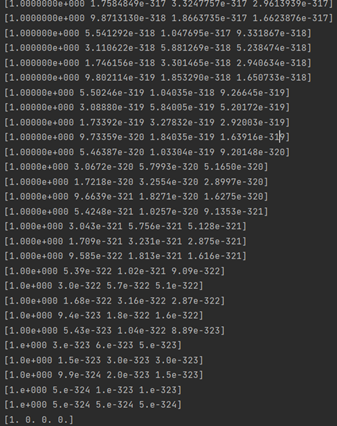3 非理想结果

（为 1），其他网站的权威值为 0 的情况，在这里我把它称为“采集器陷阱”。

四、两大常见问题和解决方法

（二）解决方法—— PageRank 的一般定义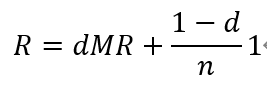1 要么以概率 d 决定按照超链接随机跳转，这时所有浏览者以该网页中存在

2 要么以概率（1-d)决定完全随机跳转，这时以等概率 1/n 跳转到任意一个

（三）案例再分析

1 单步矩阵相乘

import numpy as np
d=0.85
a=np.array([[1,1/2,1/2,1/3],[0,0,0,1/3],[0,1/2,0,1/3],[0,0,1/2,0]])
b=np.array([1/4,1/4,1/4,1/4])
R=dnp.dot(a,b)+(1-d)/4np.ones(4)#增加以 d 为基础的完全随机概率的矩阵
print®

2 设计迭代相乘

import numpy as np
d=0.85
a=np.array([[1,1/2,1/2,1/3],[0,0,0,1/3],[0,1/2,0,1/3],[0,0,1/2,0]])
b=np.array([1/4,1/4,1/4,1/4])
while 1:
R=d*np.dot(a,b)+(1-d)/4*np.ones(4)#增加以 d 为基础的完全随机概率的矩print(R)
b=R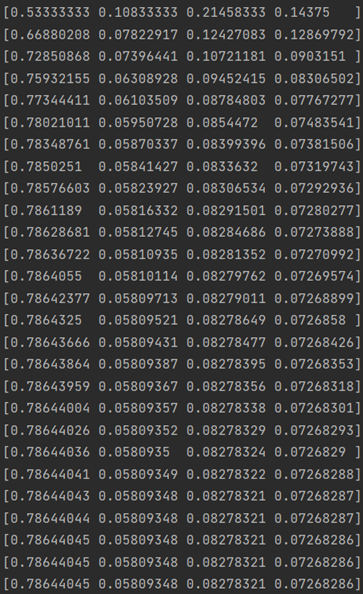五、算法设计与优化

（一）初始算法设计

1 有向图和状态转移矩阵

（1）需求分析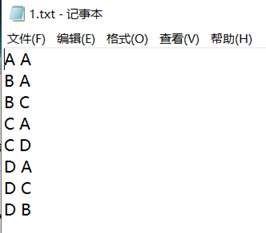（3）代码设计
import numpy as np# 读入有向图，存储边
edges=[]
f = open('1.txt', 'r')
for line in f:
edges.append(line.strip('\n').split(' '))
print(edges)# 根据边获取节点的集合
nodes = []
for edge in edges:
if edge not in nodes:
nodes.append(edge)
if edge not in nodes:
nodes.append(edge)
print(nodes)# 获取节点出链的个数
sides=dict()
for edge in edges:
if edge not in sides:
sides[edge]=1
elif edge in sides:
sides[edge] += 1
print(sides)
#序号转化
edges_num=[]
for edge in edges:
line=[]
for i in edge:
if i=='A':
i=0
elif i=='B':
i=1
elif i=='C':
i=2
elif i=='D':
i=3
line.append(i)
edges_num.append(line)
print(edges_num)
M = np.zeros([len(nodes),len(nodes)])#生成空矩阵
#循环遍历归一化
for i in edges_num:
M[i,i]=i+1
if M[i,i]==1:
M[i, i]=1/sides['A']
elif M[i,i]==2:
M[i, i]=1/sides['B']
elif M[i,i]==3:
M[i, i]=1/sides['C']
elif M[i,i]==4:
M[i, i]=1/sides['D']
print(M)
（4）输出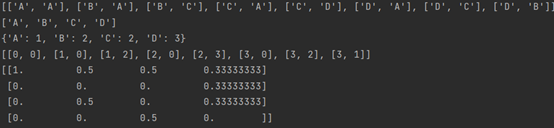2 随机游走计算 PageRank 值

import numpy as np
d=0.85
a=np.array([[1,1/2,1/2,1/3],[0,0,0,1/3],[0,1/2,0,1/3],[0,0,1/2,0]])
b=np.array([1/4,1/4,1/4,1/4])
while 1:
R=d*np.dot(a,b)+(1-d)/4*np.ones(4)#增加以 d 为基础的完全随机概率的矩print(R)
b=R

3 预期误差达到平稳分布

（3）代码设计
import numpy as np
d=0.85
a=np.array([[1,1/2,1/2,1/3],[0,0,0,1/3],[0,1/2,0,1/3],[0,0,1/2,0]])
b=np.array([1/4,1/4,1/4,1/4])
e=1
while e > 0.00000001:
R=d*np.dot(a,b)+(1-d)/4*np.ones(4)#增加以 d 为基础的完全随机概率的矩print(R)
e=R-b
e= max(map(abs, e))
b=R

（二）算法优化设计

3 代码重设计
import numpy as np# 读入有向图，存储边
edges=[]
f = open('1.txt', 'r')
for line in f:
edges.append(line.strip('\n').split(' '))
print(edges)
nodes = []
sides=dict()
for edge in edges:
edge = ord(edge) - 65
edge = ord(edge) - 65
# 根据边获取结点的集合
if edge not in nodes:
nodes.append(edge)
if edge not in nodes:
nodes.append(edge)
# 根据边获取边的字典
if edge not in sides:
sides[edge] = 1
elif edge in sides:
sides[edge] += 1
print(nodes)
print(edges)
print(sides)
M = np.zeros([len(nodes),len(nodes)])#生成空矩阵
#循环遍历归一化
for i in edges:
M[i,i]=i+1
for k in range(len(nodes)):
if M[i,i]==k+1:
M[i, i]=1/sides[k]
print(M)

（三）最终成果

1 最终代码

import numpy as np# 读入有向图，存储边
edges=[]
f = open('1.txt', 'r')
for line in f:
edges.append(line.strip('\n').split(' '))
print(edges)
nodes = []
sides=dict()
for edge in edges:
edge = ord(edge) - 65
edge = ord(edge) - 65
# 根据边获取结点的集合
if edge not in nodes:
nodes.append(edge)
if edge not in nodes:
nodes.append(edge)
# 根据边获取边的字典
if edge not in sides:
sides[edge] = 1
elif edge in sides:
sides[edge] += 1
print(nodes)
print(edges)
print(sides)
M = np.zeros([len(nodes),len(nodes)])#生成空矩阵
#循环遍历归一化
for i in edges:
M[i,i]=i+1
for k in range(len(nodes)):
if M[i,i]==k+1:
M[i, i]=1/sides[k]
print(M)
print("开始计算 PR 值......")
d=0.85
page_num = len(nodes)
#计算初始分布向量 R
R0 = np.ones(page_num)
for i in range(page_num):
R0[i]=1/page_num
e=1
while e > 0.00000001:
R=d*np.dot(M,R0)+(1-d)/4*np.ones(4)#增加以 d 为基础的完全随机概率的

print(R)
e=R-R0
e= max(map(abs, e))
R0=R

2 结果截图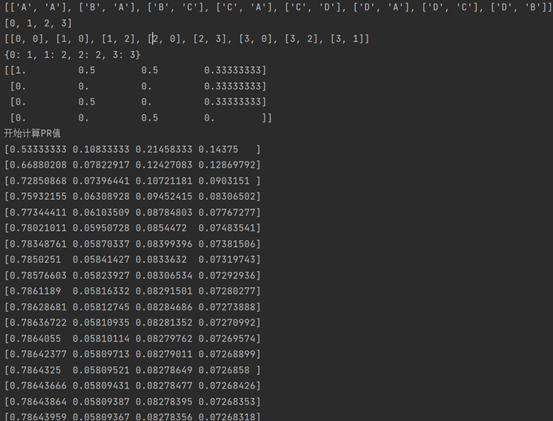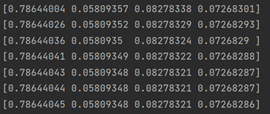3 总结与体会参考文献

 郑华,孙宇锋. PageRank 思想在若干数学课程教学中的渗透[J]. 教育教学论坛, 2020,
476(30): 294-295.
 同济大学数学系编. 工程数学 线性代数 第 6 版[M]. 北京：高等教育出版

 李航著. 统计学习方法 第 2 版[M]. 北京：清华大学出版社, 2019.05.
 清华大学《统计学习方法》第 2 版课件
 原理参考：https://blog.csdn.net/gamer_gyt/article/details/47443877
 初始算法参考：https://blog.csdn.net/ten_sory/article/details/80927738
 PageRank 算法研究现状与展望